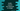# Kotlin program to convert one character to integer## Introduction :

In this tutorial, I will show you how to convert one character to integer in Kotlin. Kotlin provides a lot of utility functions for each class.

### Method 1: Using Character.getNumericValue(char ch) :

This method takes one character as a parameter and returns its integer value.

``````fun main() {
val givenNumbers = charArrayOf('0', '1', '8', '7', '9')

givenNumbers.forEach {
println("\$it => \${Character.getNumericValue(it)}")
}
}
``````

It will print the below output :

``````0 => 0
1 => 1
8 => 8
7 => 7
9 => 9
``````

We are converting each character of the character array givenNumbers.

### Method 2: By converting it to a string :

Character class provides one method toInt, but it returns the ASCII value. But if we convert the character to a string and use the toInt method defined in the string class, it will convert that string to an integer.

Let’s check the below example :

``````fun main() {
val givenNumbers = charArrayOf('0', '1', '8', '7', '9')

givenNumbers.forEach {
println("\$it => \${it.toString().toInt()}")
}
}
``````

It will print the same output as the above example.